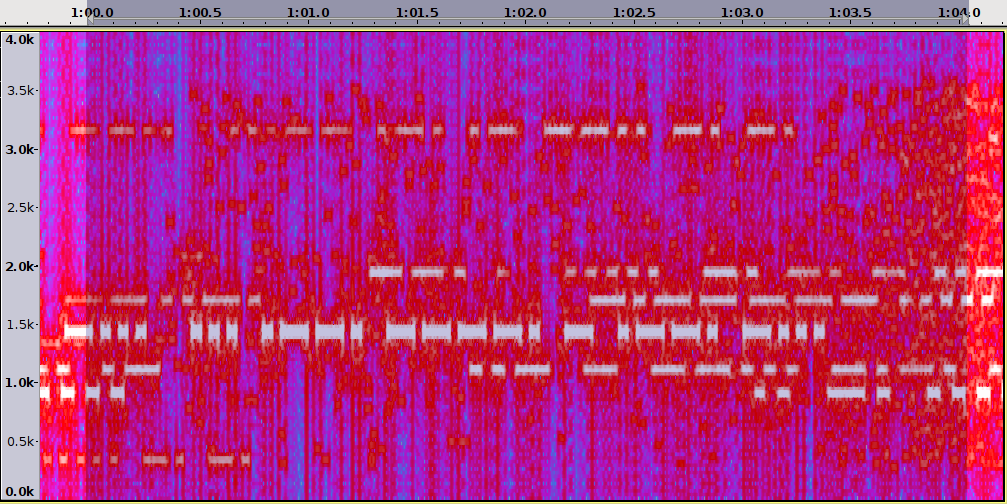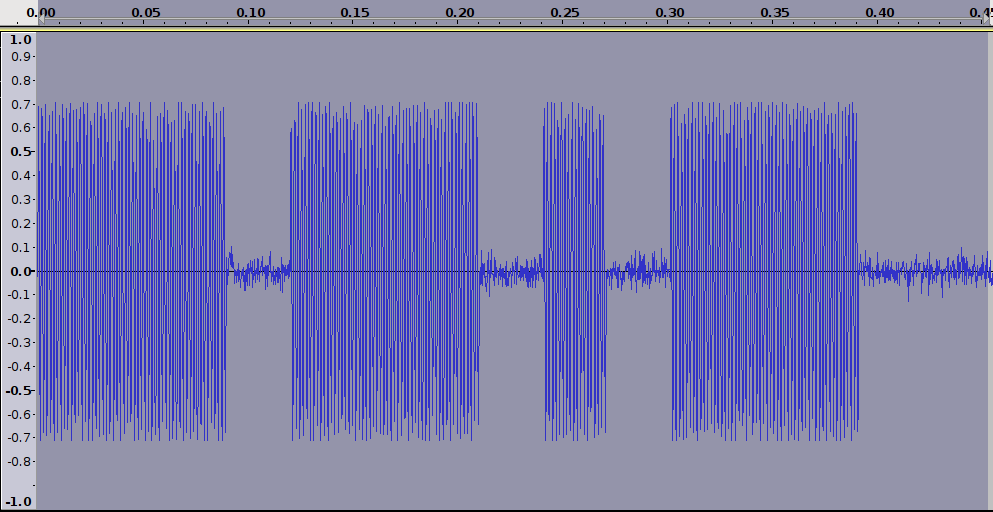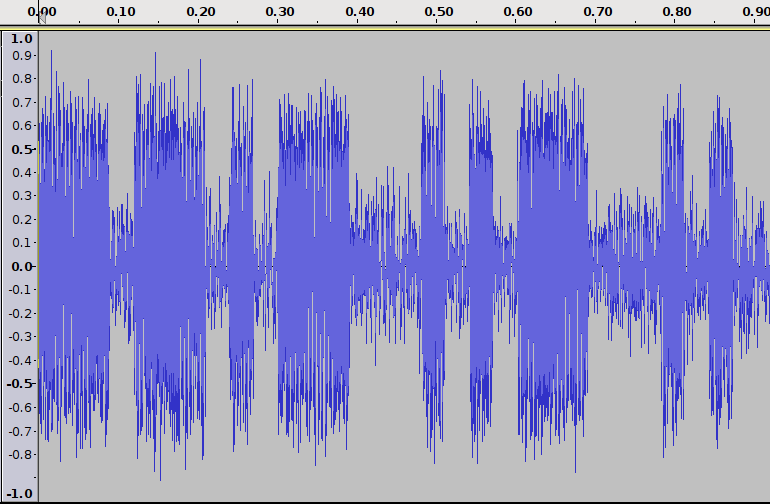## MATCHING PURSUIT METHOD FOR NOISE REDUCTION

In my previous post I shared a new method for reducing noise from Morse signals based on MPTK toolkit.  I contacted Dr. Remi Gribonval who is one of the authors of the MPTK toolkit and asked for his advice in how to optimize the atoms for Morse code signals.   To my surprise he responded the same day and gave me some great ideas. There was also a fruitful discussion in eham.net  that helped me to figure out how to optimize the dictionary suitable for processing audio signals containing Morse code.

### FREQUENCY DOMAIN VIEW

I used the same pileup.wav file than in the previous post and used the new, revised dictionary by issuing the following commands

mpd -D dic_mdct_morse2.xml -s 10 pileup.wav pileup.bin
mpr pileup.bin pileup_reco.wav

Runtime of mpd command was 73 seconds on Thinkpad X301 laptop. The length of pileup.wav is 71.5 seconds, so mpd can decompose audio almost in real time to selected 10 dB SNR.

To illustrate the noise reduction capability I zoomed into a four second long audio segment starting at 1:00.0.  The result is shown in figure 1 spectrum plot below.  Figure 2 shows the original noisy signal. It is easy to observe that there has been quite significant noise reduction and Morse signals are much more visible from background noise.  Unlike a normal bandpass filter that passes only the frequency of a single CW signal within range, this new method automatically detects and passes all CW signals in a broad frequency range while reducing surrounding noise. You may want to download the filtered audio file to listen and compare with the original audio file.Fig 1. Filtered pileup signalFig 2. Original pileup signal

Here is the content of the dictionary file  dic_mdct_morse2.xml.  Sampling rate used in the original audio file was 8000 Hz.

<?xml version="1.0" encoding="ISO-8859-1"?>
<dict>
<libVersion>0.2</libVersion>
<block>
<param name="blockOffset" value="0" />
<param name="fftSize" value="256" />
<param name="type" value="mdst" />
<param name="windowLen" value="256" />
<param name="windowShift" value="32" />
<param name="windowopt" value="0" />
<param name="f0Max" value="800" />
<param name="f0Min" value="400" />
<param name="windowtype" value="rectangle" />
</block>

<block>
<param name="blockOffset" value="0" />
<param name="fftSize" value="256" />
<param name="type" value="mdst" />
<param name="windowLen" value="256" />
<param name="windowShift" value="64" />
<param name="windowopt" value="0" />
<param name="f0Max" value="800" />
<param name="f0Min" value="400" />
<param name="windowtype" value="rectangle" />
</block>

</dict>

### TIME DOMAIN VIEW

I wanted to see how this new noise reduction method impacts the amplitude envelope of the CW signals. I created a sample Morse audio test file with 20 dB SNR and used a dictionary file dic_mdct_morse.xml below for processing.   Figure 3. below shows letter Q  - as you can observe there is visible noise riding on signal and also between "dahs" and "dit".Fig 3.  Letter Q  with 20 dB SNR

Figure 4. below shows filtered signal.  The waveform shows no noise between "dahs" and "dit".  Also, there is very little noise on signal but selected atoms are visible as 3 "bumps" riding on top of "dah".Fig 4. Letter Q filtered

I did another experiment with much worse signal-to-noise ratio.  Figure 6. shows letters QUI  with 6 dB SNR  (this was normalized to -3 dB before processing to prevent overflows).  Figure 5 shows the filtered version of this signal. Noise reduction is visible but there is still some noise left.Fig 5.  Filtered QUIFig 6.  Letters QUI with 6 dB SNR

Here is the dictionary file  dic_mdct_morse.xml used in the time domain examples above.  The sampling rate in these artificially generated files is 4000 Hz therefore the atom sizes are scaled down.

<?xml version="1.0" encoding="ISO-8859-1"?>
<dict>
<libVersion>0.2</libVersion>
<block>
<param name="blockOffset" value="0" />
<param name="fftSize" value="128" />
<param name="type" value="mdst" />
<param name="windowLen" value="128" />
<param name="windowShift" value="16" />
<param name="windowopt" value="0" />
<param name="f0Max" value="800" />
<param name="f0Min" value="400" />
<param name="windowtype" value="rectangle" />
</block>

<block>
<param name="blockOffset" value="0" />
<param name="fftSize" value="128" />
<param name="type" value="mdst" />
<param name="windowLen" value="128" />
<param name="windowShift" value="32" />
<param name="windowopt" value="0" />
<param name="f0Max" value="800" />
<param name="f0Min" value="400" />
<param name="windowtype" value="rectangle" />
</block>

</dict>

### CONCLUSIONS

Matching Pursuit is a powerful new digital audio processing method for reducing noise in Morse signals.  The experiments above demonstrate that it is possible to significantly improve signal to noise ratio even in cases where there are multiple CW stations sending simultaneously at nearby frequencies.
MPTK toolkit provides rich set of tools to further optimize the digital signal processing. Examples above were the result of some 3 hours of work testing various atoms and related parameters.

All software used is available as open source making it feasible to integrate this noise reduction method to various other projects.  If you find this article valuable please provide your comments below.

73
Mauri AG1LE

1.2.